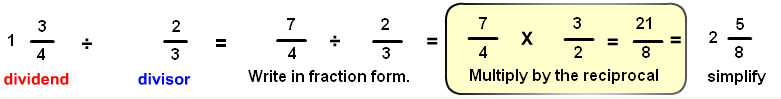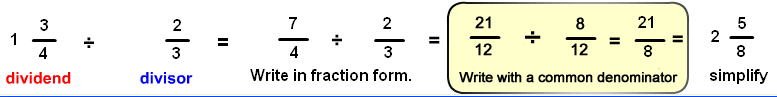# Divide with number line

## Learn or teach by designing your own divide visual fractions examples. All examples are modeled with number lines.

÷
dividend
divisor
DIVIDE WITH NUMBER LINE MODELS
SHOW COLOR
EXPLAIN
SHOW INPUT

# INSTRUCTIONS

With Divide With Number Line Models Designer you can design fractions examples that use number line models to demonstrate dividing fractions .

You can input the divisor and dividend for a division of fractions example. The dividend or divisor each must be less than 7. The quotient must be less than 20.

If you want just a whole number for for the divisor or the dividend, type in 0 (zero) for a numerator. If you do not want a whole number, type in 0 (zero) for the whole number. Do not type in 0 for the denominator.

Think of the dividend as the length of cake and the divisor as the length of a container. If the cake is 5 12 units in length and each container pan can hold 34 of a unit, you can fill 7 containers with one piece left over. The piece will fill 13 of a container so you can fill 7 13 containers.

Visualizing division examples is not easy. You are told that division by a number can be done by multiplying by the reciprocal of that number:;

Another method would be to write the dividend and divisor over a common denominator. With like fractions you can divide the dividend numerator with the divisor numerator. For example:This method is not the easiest but it helps us to see the relative sizes of the dividend and divisor.

You can then compare this with the image for the same example. Notice the dividend has 21 common denominator marks and the divisor has 8 common denominator marks giving 218 for the quotient.

So with division you are asking how much or how many of the divisor size will fit into the dividend.

Uncheck the <EXPLAIN> check box to turn off the answer and the explanation. You can ask your learners to complete the number sentence.

Uncheck the <SHOW INPUT> check box to make the input dialog boxes work like a password input boxes, hiding the numbers you input.

With <EXPLAIN> and <SHOW INPUT> unchecked you can ask your learners to write a number sentence that explains the picture.

With <EXPLAIN> and <SHOW COLOR> unchecked you can ask your learners to complete the picture by shading the circles.

Suggestions:

Start with a dividend of 2 14 and a divisor of 23. Notice how 3 38 divisor amounts fit into the dividend. Increase the divisor by 1 to get 2 14 divided by 1 23 giving a quotient of 1 720. Then increase the divisor to 2 23. This will result in a quotient smaller than one (2732) , showing that the quotient is smaller than one when the divisor is larger than the dividend.

You can also demonstrate how as the divisor decreases the quotient increases. Try a Dividend of 2 23 and a divisor of 2 23. Notice that the divisor and dividend are the same size, resulting in a quotient of one. Decrease the divisor by 13 increments. For example, keep the dividend at 2 23 and change the divisor to 2 13, 2 03, 1 23, etc, and continue with this pattern. You will see the quotient increase.

Try 1 34 divided by 3 12. Notice that only half the divisor fits into the dividend.

Divide one(1) by 23. (Enter 1 for the whole number, 0 for the numerator and 1 for the denominator. Then enter 0 and 23 for the divisor. You will get 32 or 1 12 for the quotient. This shows that 1 divided by any fraction will give the reciprocal (inverse) of the fraction.

Think of the dividend as available pizza and the divisor as the amount of the available pizza that can fit into a take-out container. If there are 5 12 pizzas and the container can hold 3/4 pizza, you can fill 7 containers with one piece left over. The piece will fill 1/3 of a container, so you can fill 7 1/3 containers.

How do you explain what's really going on when you divide 12 by 23? This is hard to picture, but if you cover the dividend 12 by the divisor 23 only 34 of the divisor is needed to cover the dividend. If you cover 23 by 12 you will find it will take a whole 12 piece plus 13 of the 12 piece.

WINDOWS COMPUTERS

Windows users can select any part of the screen by right clicking and selecting "Take a screenshot". Adjust to fit mage you want. This copies the selection into Windows Clipboard™. The screen can then be pasted into Windows Paint™ or your favorite imaging program. Or you can select "Download" which will put the image into your files "Download" folder.